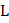# The graph between kinetic energy and momentum of a particle is plotted as shown in fig. The mass of the moving particle isa) 1kg b) 2kg c) 3kg d) 4kg

## Question ID - 100214 :- The graph between kinetic energy and momentum of a particle is plotted as shown in fig. The mass of the moving particle isa) 1kg b) 2kg c) 3kg d) 4kg

3537

 (b)From the graph,Next Question :

Three identical uniform rods of the same massand lengthare arranged inplane as shown in fig. A fourth uniform rod of mass 3has been placed as shown in theplane. What should be the value of the length of the fourth rod such that the centre of mass of all the four rods lie at the origin?a) 3b) 2c)(+1)/3 d)(2+1)/3